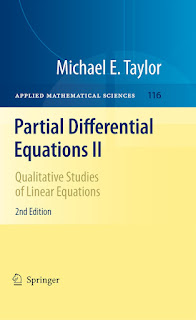ANALYSICS MATHEMATICS

# Partial Differential Equations II Qualitative Studies of Linear Equations PDF Free DownloadThis second in the series of three volumes builds upon the basic theory of linear PDE given in volume 1, and pursues more advanced topics. Analytical tools introduced here include pseudodifferential operators, the functional analysis of self-adjoint operators, and Wiener measure. The book also develops basic differential geometrical concepts, centered about curvature. Topics covered include spectral theory of elliptic differential operators, the theory of scattering of waves by obstacles, index theory for Dirac operators, and Brownian motion and diffusion. The book is targeted at graduate students in mathematics and at professional mathematicians with an interest in partial differential equations, mathematical physics, differential geometry, harmonic analysis, and complex analysis.In this second edition, there are seven new sections including Sobolev spaces on rough domains, boundary layer phenomena for the heat equation, the space of pseudodifferential operators of harmonic oscillator type, and an index formula for elliptic systems of such operators. In addition, several other sections have been substantially rewritten, and numerous others polished to reflect insights obtained through the use of these books over time.